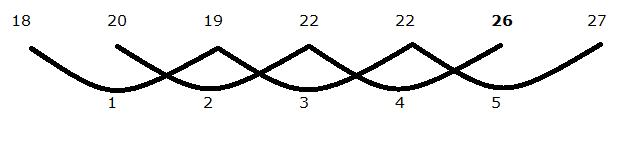# gkrecall

We brings you daily Current affairs, daily Current Affairs Quiz, weekly Current Affairs, weekly Current Affairs Quiz to enhance your preparation for upcoming exams. We also provide articles related to quant, reasoning, English, ssc etc .

## Breaking

We bring you the complete and important daily Quant quiz  to achieve more marks in Banking, Insurance, UPSC, SSC, CLAT, Railways and all other competitive Exams. We prepare it based on our daily current affairs.Hope you like it.

Web

Wrong Number Series
Directions (Q. 1 – 5): Find the wrong term in the following number series?
1) 4, 12, 60, 360, 2520, 20160
a) 4
b) 2520
c) 20160
d) 60
e) 360
2) 90, 86, 95, 79, 103, 68, 117
a) 86
b) 79
c) 95
d) 103
e) 117
3) 11, 12, 25, 76, 304, 1526, 9157
a) 25
b) 304
c) 1526
d) 11
e) 9157
4) 18, 20, 19, 22, 22, 24, 27
a) 18
b) 27
c) 24
d) 20
e) 19
5) 1.5, 9, 24, 96, 384, 1536, 6144
a) 96
b) 6144
c) 24
d) 384
e) 9
Data Interpretation
Directions (Q. 6 – 10): Study the following information carefully and answer the given questions?
The following table shows the percentage distribution of total students participated in different sports (Badminton, Cricket and Volleyball) from different schools in the year 2017 and the remaining students didn’t participated in any games. Some values are missing here.
Note:
Total number of students participated in Badminton, Cricket and Volleyball is not equal to the total number of students studied in those schools. Some students are not interested in sports.
6) Find the difference between the total number of students not participated in any sports from school A to that of school C, if the total number of students participated in Volleyball from school A is 256, which is equal to 16 % of total students in that school and the percentage of students participated in Cricket from school C is 17 %?
a) 82
b) 70
c) 43
d) 55
e) None of these
7) Find the average number of students studied in all the given schools together, if the total number of students participated in Volleyball in school A is 256 and the percentage of total number of students not participated in any sports from school A is 32 % and the total number of students participated in Cricket from school D is 240?
a) 1460
b) 1580
c) 1620
d) 1740
e) None of these
8) Total number of students participated in badminton from school C and E together is approximately what percentage of total number of students participated in Cricket from school A and B together, if the total number of students participated in Badminton from school A is 384?
a) 97 %
b) 146 %
c) 156 %
d) 114 %
e) 125 %
9) Find the ratio between the total number of students participated in Badminton from school A and D together to that of total number of students participated in Volleyball from school B and C together, if the total number of students participated in Cricket from school A is 448 and the ratio between the total number of students in school B to that of D is 6 : 5?
a) 72 : 59
b) 218 : 185
c) 11 : 5
d) 45 : 31
e) None of these
10) Total number of students studied in school E is approximately what percentage more/less than the total number of students participated in Cricket from school B and C together, if the percentage of total number of students not participated in any sports from school C is 37 %?
a) 200 % more
b) 200 % less
c) 325 % more
d) 325 % less
e) 275 % more

Directions (1-5):
3 * 4 = 12
12 * 5 = 60
60 * 6 = 360
360 * 7 = 2520
2520 * 8 = 10160
The wrong term is, 4
90,              86,              95,              79,              104,            68,          117
-4                +9               -16              -25              +36             -49
The difference is, – 22, + 32, – 42, – 52, – 62, – 72,…..
The wrong term is, 103
11 * 1 + 1 = 12
12 * 2 + 1 = 25
25 * 3 + 1 = 76
76 * 4 + 1 = 305
305 * 5 + 1 = 1526
1526 * 6 + 1 = 9157
The wrong term is, 304
The wrong term is, 241.5 * 4 = 6
6 * 4 = 24
24 * 4 = 96
96 * 4 = 384
384 * 4 = 1536
1536 * 4 = 6144
The wrong term is, 9
Directions (6-10):
The total number of students not participated in any sports from school A
= > 256 * (100/16) * (32/100) = 512
The total number of students not participated in any sports from school C
= > 1500 * (37/100) = 555
Required difference = 555 – 512 = 43
The average number of students studied in all the given schools together
= > [(256/16) * 100 + 1200 + 1500 + (240/24) * 100 + 2000] / 5
= > [1600 + 1200 + 1500 + 1000 + 2000] / 5
= > [7300 / 5] = 1460
Total number of students participated in badminton from school C and E together
= > 1500 * (25/100) + 2000 * (30/100)
= > 375 + 600 = 975
Total number of students participated in Cricket from school A and B together
= > (384 / 24) * 100 * (28/100) + 1200 * (18/100)
= > 448 + 216 = 664
Required % = (975 / 664) * 100 = 146.83 % = 146 %